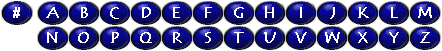This index covers the introductions to the rules, the rules, and Appendices A, C, and E.  All other appendices are indexed by title only.  Neither the examples nor works cited in any of the rules or appendices are indexed.  The Glossary is not indexed.  Specific marks of punctuation are not usually indexed, although there are some exceptions, such as brackets and dashes.

Most entries refer to rule numbers.  The general introduction is indexed by the abbreviation "Intro."  An introduction to a chapter is indexed by the chapter number, followed by "intro.," e.g., Ch. 2, intro.  An introduction to a rule is indexed by the rule number, followed by "intro.," e.g., 1G, intro.

This index is arranged word-by-word, and commas and hyphens in the headings are ignored for filing purposes.

Rerelease titles

See Rerelease--

titles

Abbreviations used in this index are:

 App. Appendix Ch. Chapter Intro. Introduction to the manual intro. Introduction to a chapter or a section